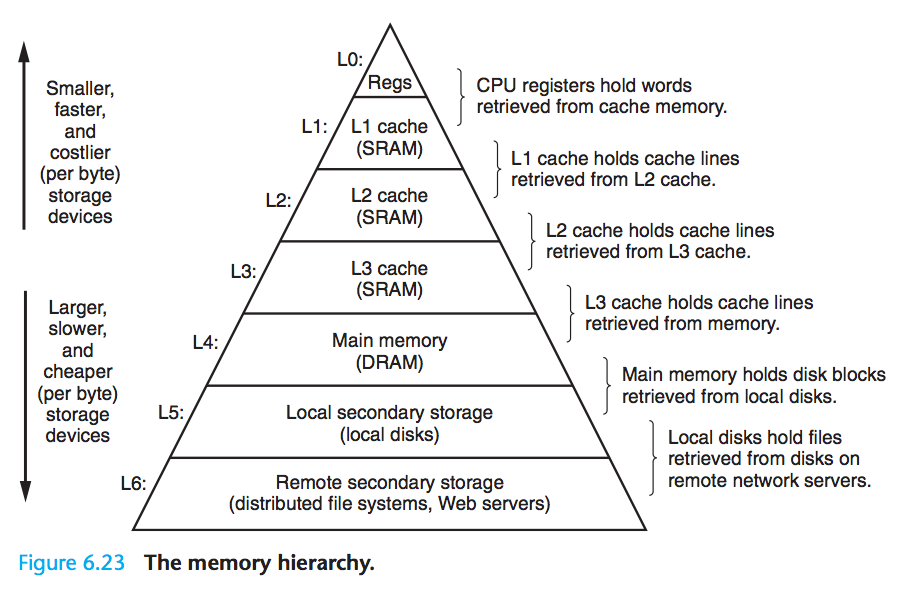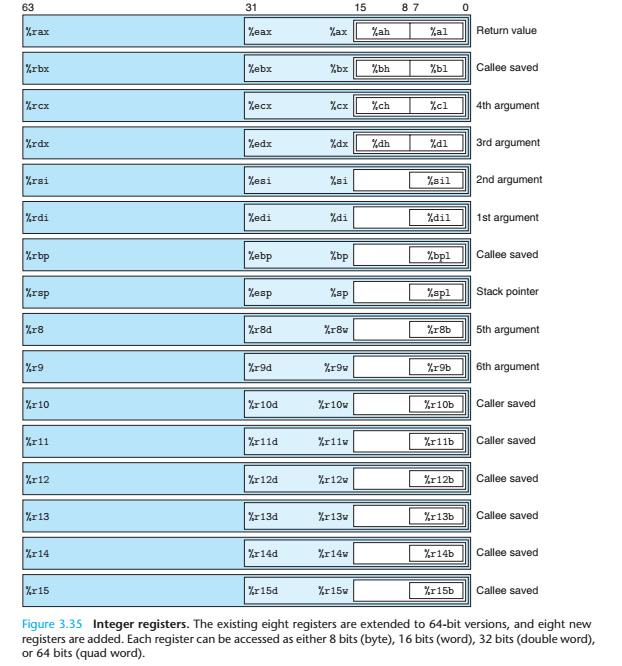book

flag
mode_edit

• +==
• *(a + i)a[i]
• x * 10(x << 1) + (x << 3)
• 循环中，++ii++
• 循环变量加上register
• std::pair <T1, T2>很慢
• std::minstd::max很慢
• if ... else ......?...:...

## 卡常的一套理论

### 存储器层次结构

#### 存储器层次结构L1 高速缓存64 字节块芯片上的 L1 高速缓存4硬件（反正你控制不了）
L2 高速缓存64 字节块芯片上的 L2 高速缓存10硬件（同上）
L3 高速缓存64 字节块芯片上的 L3 高速缓存50硬件（同上）

(CPU 周期：CPU 频率的倒数)

### 程序的机器级表示

#### 寄存器

x86-64的寄存器充分体现了它的历史遗迹：#### 操作数指示符

• 间接寻址：用寄存器里的值作为内存地址（比如这个寄存器里放了一个指针）：(%rxx)，也就是把寄存器用括号括起来
• 基址+偏移量寻址：用寄存器里的值加上一个立即数作为内存地址：Imm(%rxx)，得到的结果是Memory[%rxx + Imm]
• 比例变址寻址（这个名字听起来很牛逼）Imm(%rxx, %ryy, s)，得到的结果是Memeory[Imm + %rxx + %ryy * s]，其中，比例因子 $s$ 只能是 $1,\ 2,\ 4,\ 8$

#### 常见指令

• mov S D，作用：S -> D
• lea S D，作用：&S -> D
• inc D，作用：D + 1 -> D
• dec D，作用：D - 1 -> D
• neg D，作用：-D -> D
• add S D，作用：D + S -> D
• sub S D，作用：D - S -> D
• imul S D，作用：D * S -> D
• xor S D，作用：D ^ S -> D
• sal k D，作用：D << k -> D
• sar k D，作用：D << k -> D（算术右移）
• shr k D，作用：D << k -> D（逻辑右移）

### 优化的思路

#### 消除不必要的内存引用

#define Lowbit(x) (x&-x)
void Change2(register int k,const int & x) {
while (k > 0) {
f[k] += x;
k -= Lowbit(k);
}
}


#### 编写高速缓存友好的代码

##### 提高时空局部性

int siz[maxn], top[maxn], son[maxn], fa[maxn], dep[maxn];


struct node
{
int siz, top, son, dep, fa;
node() { siz = top = dep = fa = 0;}
};
node nd[maxn];


## 做一做实验

### +=与=

int func (int & a, int b) {
a += b;
return a;
}
int func2 (int & a, int b) {
a = a + b;
return a;
}


### *(a + i)与a[i]

int vec[(int)1e3];
int func (unsigned int idx) {
return vec[idx];
}
int func2 (int idx) {
return vec[idx];
}
int func3 (unsigned int idx) {
return *(idx + vec);
}
int func4 (int idx) {
return *(idx + vec);
}



### x * 10与(x << 1) + (x << 3)

int func(int x) {
return x * 10;
}
int func2(unsigned int x) {
return x * 10;
}
int func3(int x) {
return (x << 1) + (x << 3);
}
int func4(unsigned int x) {
return (x << 1) + (x << 3);
}


leal    (%rdi,%rdi,4), %eax


2 * (x + x * 4)，指令条数和移位版本还是相同的。

### ++i与i++

int vec[(int)1e3];
int func (int n) {
int sum = 0;
for (int i = 0; i < n; ++i)
sum += vec[i];
return sum;
}
int func1 (int n) {
int sum = 0;
for (int i = 0; i < n; i++)
sum += vec[i];
return sum;
}


### register有用吗

int vec[(int)1e3];
int func (register int n) {
int sum = 0;
for (register int i = 0; i < n; ++i)
sum += vec[i];
return sum;
}
int func1 (int n) {
int sum = 0;
for (int i = 0; i < n; i++)
sum += vec[i];
return sum;
}


### std::min与手写版本

int mmin (int n, int m) {
if (n < m) return n;
else return m;
}

int mmin2 (int n, int m) {
return (n < m) ? n : m;
}
#include <algorithm>
int mmin3(int n, int m) {
return std::min(n, m);
}


## 拓展阅读

navigate_before navigate_next